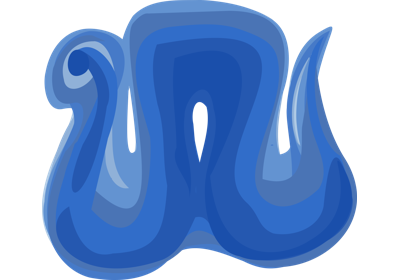# cruise_cortex_extraction¶

`nighres.cortex.``cruise_cortex_extraction`(init_image, wm_image, gm_image, csf_image, vd_image=None, data_weight=0.4, regularization_weight=0.1, max_iterations=500, normalize_probabilities=False, correct_wm_pv=True, wm_dropoff_dist=1.0, topology='wcs', topology_lut_dir=None, save_data=False, overwrite=False, output_dir=None, file_name=None)[source]

CRUISE cortex extraction

Segments the cortex from a whole brain segmented data set with the CRUISE method (includes customized partial voluming corrections and the Anatomically-Consistent Enhancement (ACE) of sulcal fundi).

Note that the main input images are generated by the nighres module `nighres.brain.extract_brain_region()`.

Parameters: init_image (niimg) – Initial white matter (WM) segmentation mask (binary mask>0 inside WM) wm_image (niimg) – Filled WM probability map (values in [0,1], including subcortical GM and ventricles) gm_image (niimg) – Cortical gray matter (GM) probability map (values in [0,1], highest inside the cortex) csf_image (niimg) – Sulcal cerebro-spinal fluid (CSf) and background probability map (values in [0,1], highest in CSf and masked regions) vd_image (niimg, optional) – Additional probability map of vessels and dura mater to be excluded data_weight (float) – Weighting of probability-based balloon forces in CRUISE (default 0.4, sum of {data_weight,regularization_weight} should be below or equal to 1) regularization_weight (float) – Weighting of curvature regularization forces in CRUISE (default 0.1, sum of {data_weight,regularization_weight} should be below or equal to 1) max_iterations (int) – Maximum number of iterations in CRUISE (default is 500) normalize_probabilities (bool) – Whether to normalize the wm, gm, and csf probabilities (default is False) correct_wm_pv (bool) – Whether to correct for WM partial voluming in gyral crowns (default is True) wm_dropoff_dist (float) – Distance parameter to lower WM probabilities away from current segmentation (default is 1.0 voxel) topology ({'wcs', 'no'}) – Topology setting, choose ‘wcs’ (well-composed surfaces) for strongest topology constraint, ‘no’ for no topology constraint (default is ‘wcs’) topology_lut_dir (str) – Path to directory in which topology files are stored (default is stored in TOPOLOGY_LUT_DIR) save_data (bool) – Save output data to file (default is False) overwrite (bool) – Overwrite existing results (default is False) output_dir (str, optional) – Path to desired output directory, will be created if it doesn’t exist file_name (str, optional) – Desired base name for output files with file extension (suffixes will be added) Dictionary collecting outputs under the following keys (suffix of output files in brackets) cortex (niimg): Hard segmentation of the cortex with labels background=0, gm=1, and wm=2 (_cruise_cortex) gwb (niimg): Gray-White matter Boundary (GWB) level set function (_cruise_gwb) cgb (niimg): CSF-Gray matter Boundary (CGB) level set function (_cruise_cgb) avg (niimg): Central level set function, obtained as geometric average of GWB and CGB (not the middle depth of the cortex, use volumetric_layering if you want accurate depth measures) (_cruise-avg) thickness (niimg): Simple cortical thickness estimate: distance to the GWB and CGB surfaces, in mm (_cruise-thick) pwm (niimg): Optimized WM probability, including partial volume and distant values correction (_cruise-pwm) pgm (niimg): Optimized GM probability, including CSF sulcal ridges correction (_cruise_pgm) pcsf (niimg): Optimized CSF probability, including sulcal ridges and vessel/dura correction (_cruise-pwm) dict

Notes

Original algorithm by Xiao Han. Java module by Pierre-Louis Bazin. Algorithm details can be found in 

References

  X. Han, D.L. Pham, D. Tosun, M.E. Rettmann, C. Xu, and J. L. Prince, CRUISE: Cortical Reconstruction Using Implicit Surface Evolution, NeuroImage, vol. 23, pp. 997–1012, 2004

## Examples using `nighres.cortex.cruise_cortex_extraction`¶Cortical depth estimation from MGDM segmentation